# 理解机器人学的Singularity

Before understanding the singularities in robotics, we shall review:

• What are rotation matrices?
• What are Euler angles?p

I think these two points are the bases of the comprehension of singularities.

# Fundamentals

## Reference frames & forward kinematics

### Forward kinematics

• 计算什么？end-effector的位置（和速度）
• 用什么算？joint parameters的指定值

Forward kinematics refers to the use of the kinematic equations of a robot to compute the position of the end-effector from specified values for the joint parameters. [bib1]

#### Planar RRR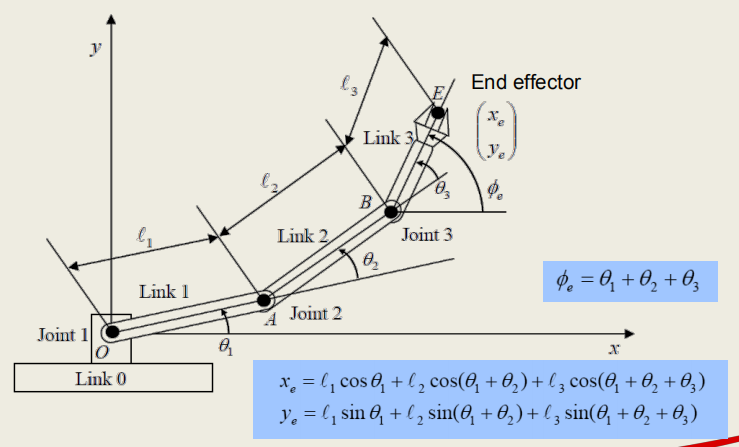• 使用joint处的相对夹角$\theta$而不是与x轴的夹角，是为了便于joint处的motor的建模

\begin{equation} \begin{aligned} x_e &= l_1cos\theta+l_2cos(\theta_1+\theta_2)+l_3cos(\theta_1+\theta_2+\theta_3)\\ y_e &= l_1sin\theta+l_2sin(\theta_1+\theta_2)+l_3sin(\theta_1+\theta_2+\theta_3) \end{aligned} \end{equation}\label{eq1}

#### Vectors

##### Left-handed & right-handedRight-handed coordinates system is more widely-used. It should be indicated in specific tutorials of a robot.

• x axis: thumb
• y axis: index finger
• z axis: middle finger

## Moving between frames

About: Rotation & Translation of frames.

### Mapping: from frame to frame

#### Translation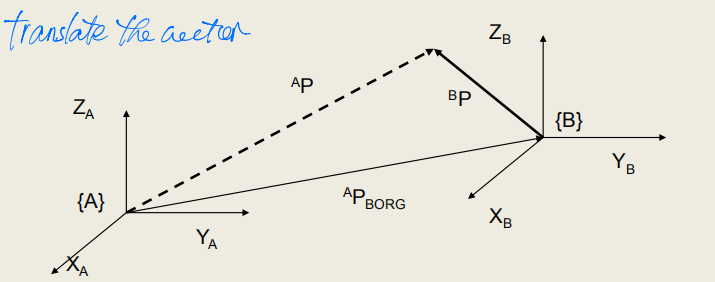#### Rotation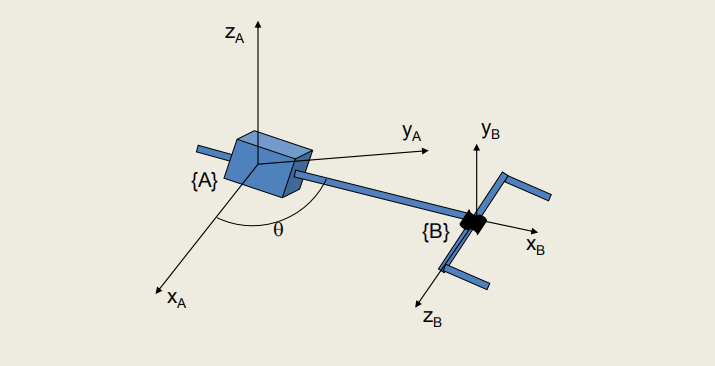Moving from axes in {A} to axes in {B}.从A系到B系的位置关系。

i.e. a description of {B} w.r.t. {A}相对于A系B系的空间位置。

##### Rotation between two frames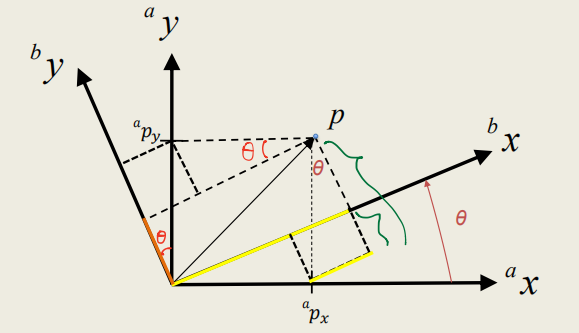$$\begin{equation} ^bp_x = ^ap_xcos\theta + ^ap_ysin\theta \\ ^bp_y = ^ap_ycos\theta - ^ap_xsin\theta \end{equation}\label{eq3}$$

#### Rotation Matrix

{b}系p点坐标作为需要求的，是从{a}系信息求的。那么反过来，用{b}系的点坐标得到相应在{a}中的坐标，见下面的公式。那么这时候{b}到{a}的旋转应理解为反向旋转$\theta$，这样公式才正确。 $$\begin{equation} ^ap_x = ^bp_xcos\theta - ^bp_ysin\theta \\ ^ap_y = ^bp_ycos\theta + ^bp_xsin\theta \end{equation}\label{eq4}$$

##### Rotation Matrix: from 2D to 3D

$$\begin{equation}^AR_B = \left[ \begin{matrix} cos\theta & -sin\theta & 0\\ sin\theta & cos\theta & 0\\ 0& 0& 1 \end{matrix} \right] \end{equation}\label{eq8}$$

##### Rotation Matrices: around x/y/z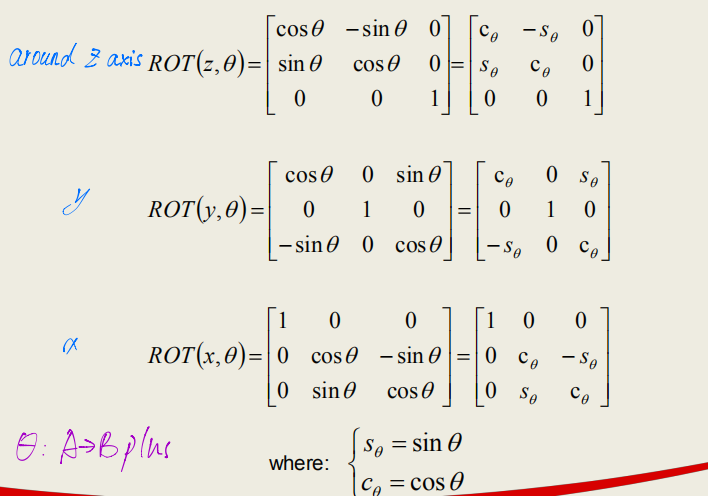Q: 这也太难记了吧？我可咋整啊？即使通过原来联立的方程组也很难算啊......

A: 我有个特殊的记忆方法：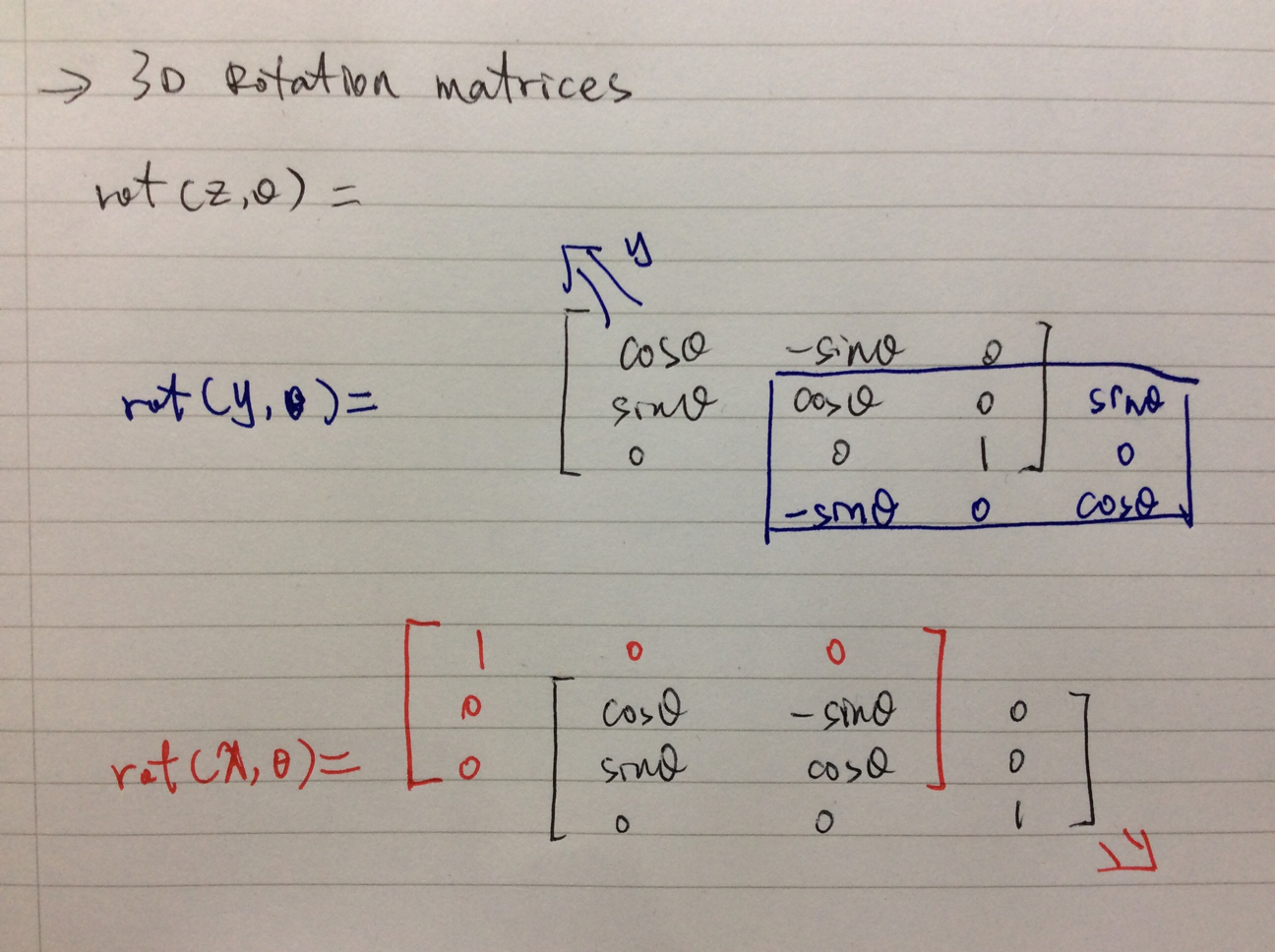##### Properties of rotation matrices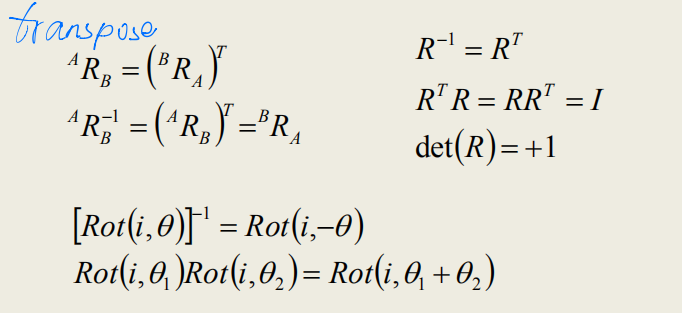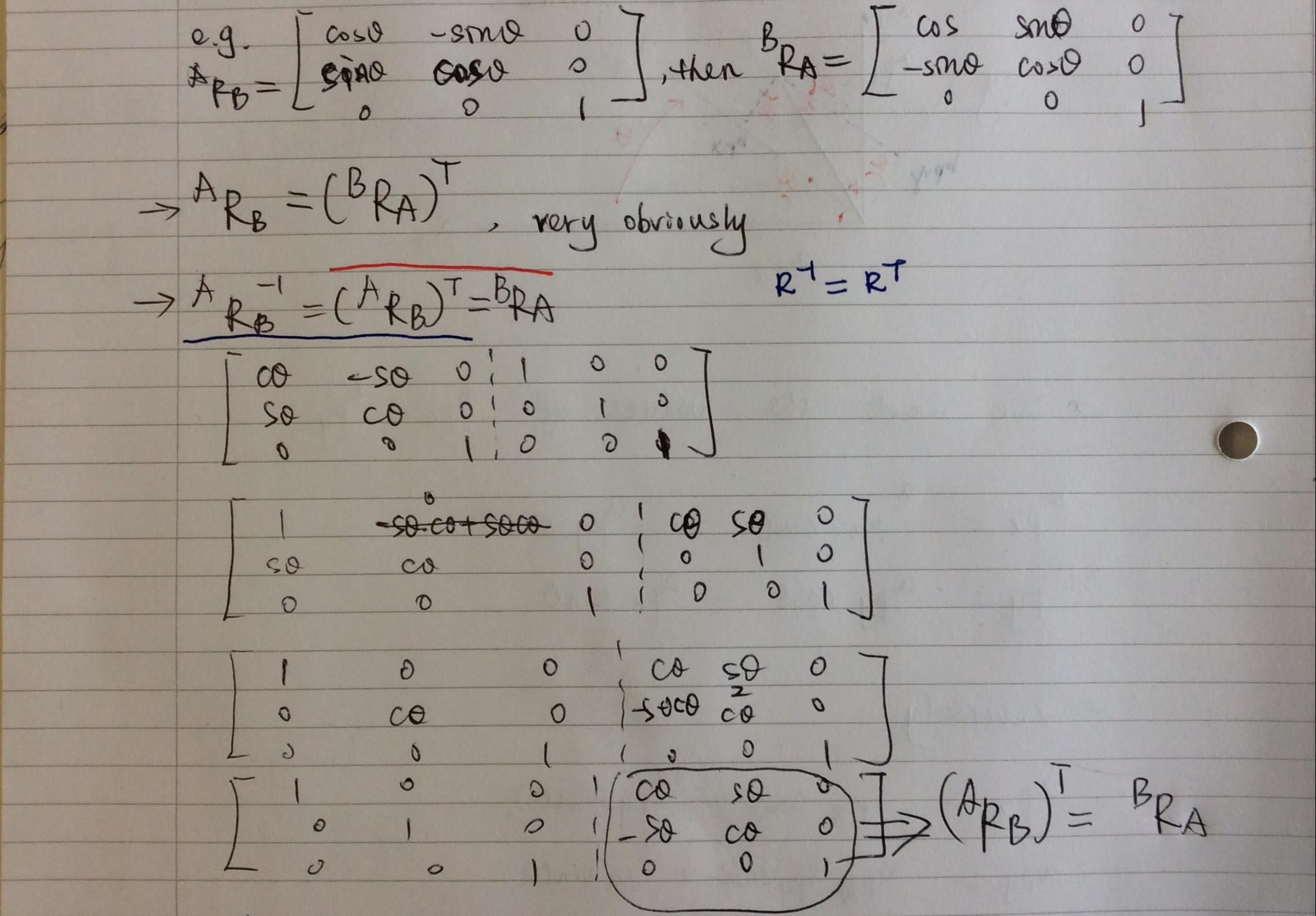🗒 还有种好记的方法：旋转矩阵的每一行就是{A}的坐标轴在{B}中的表示

## Compound Transformations

### Kinematic relationship

• 位置变换通过向量来表示
• 角度/方向变换矩阵来表示

#### 变换用矩阵表示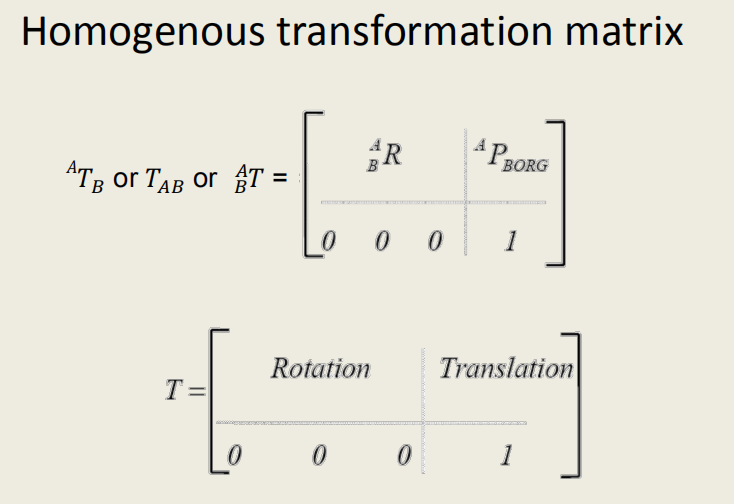• [0 0 0]是perspective transformation
• 1是scaling factor

**这个矩阵是怎么得出来的？**参考推导齐次变换矩阵

#### 逆变换矩阵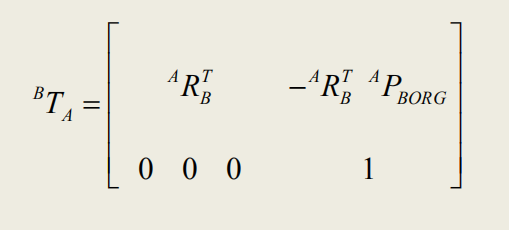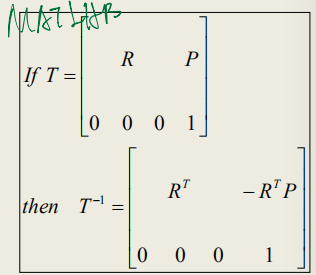#### Compound transformations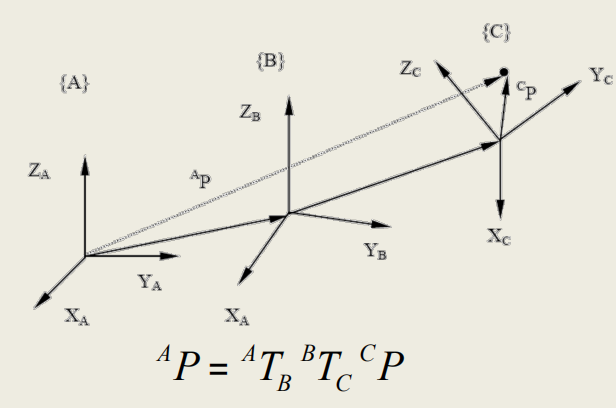##### Order matters?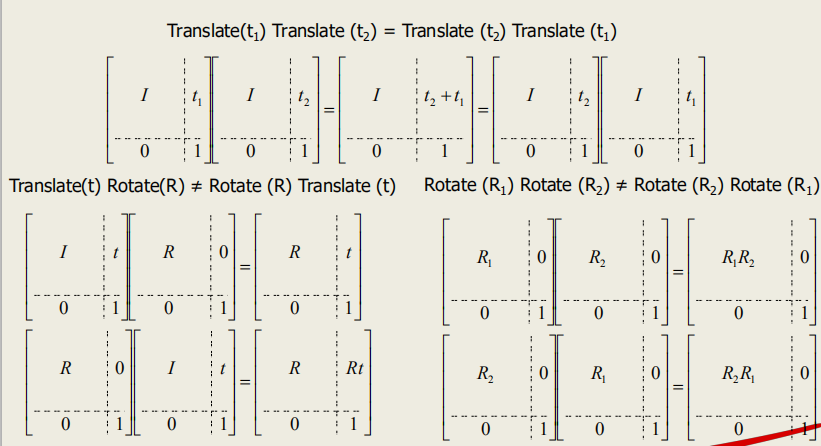#### Rotation representations

##### Unit vectors

\begin{equation} \begin{aligned} R_{ab}= \left[ \begin{matrix} x_{ab} & y_{ab} & z_{ab} \end{matrix} \right]= \left[ \begin{matrix} x_ax_b & x_ay_b & x_az_b\\ y_ax_b & y_ay_b & y_az_b\\ z_ax_b & z_ay_b & z_az_b \end{matrix} \right] \end{aligned} \end{equation}\label{eq13}

Need a proof.

##### Euler angles
• 用三个角度表示方向
• 常常按照是绕X, Y, Z轴的旋转顺序来规定
• 旋转顺序称为Euler Angle Sequence
• Minimal representation of orientation
###### 12 ways to store Euler angles

\begin{aligned} \begin{matrix} XYZ & XZY & XYX & XZX \\ YXZ & YZX & YXY & YZY \\ ZXY & \pmb{ZYX} & \pmb{ZXZ} & \pmb{ZYZ} \end{matrix} \end{aligned}

###### ZYZ: rotation matrix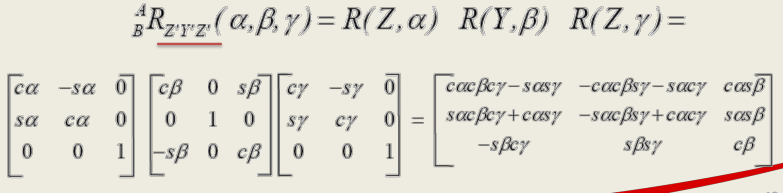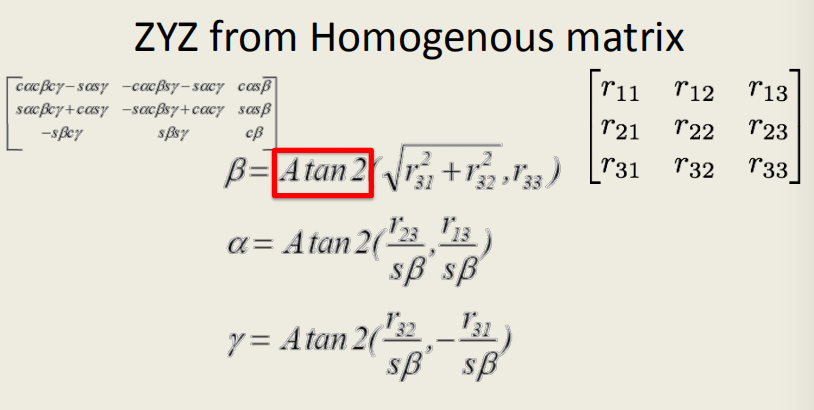# Understanding singularity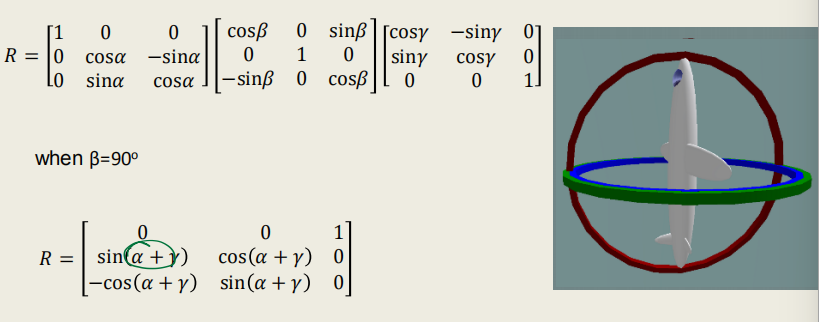• z blue torus: roll

• y green torus: yaw or heading or pan

• x red torus: pitch or tilt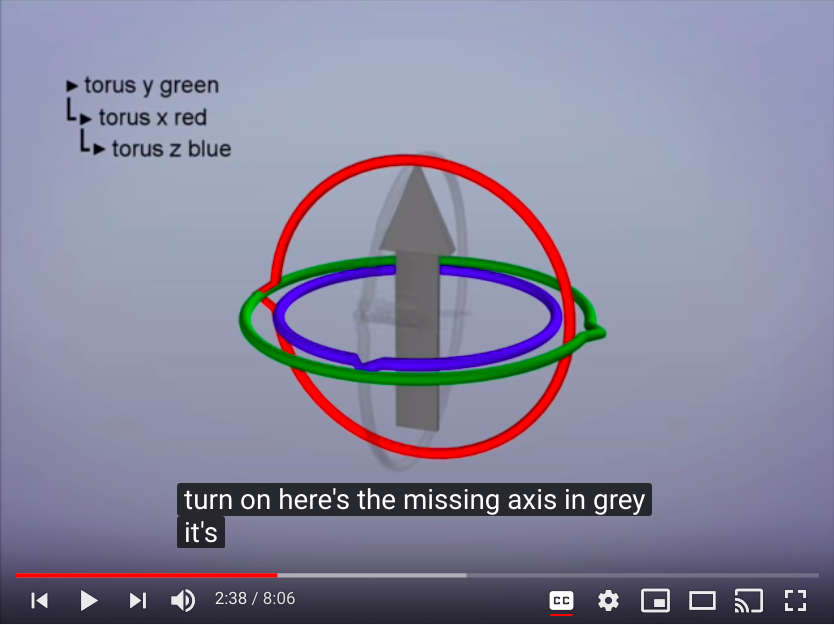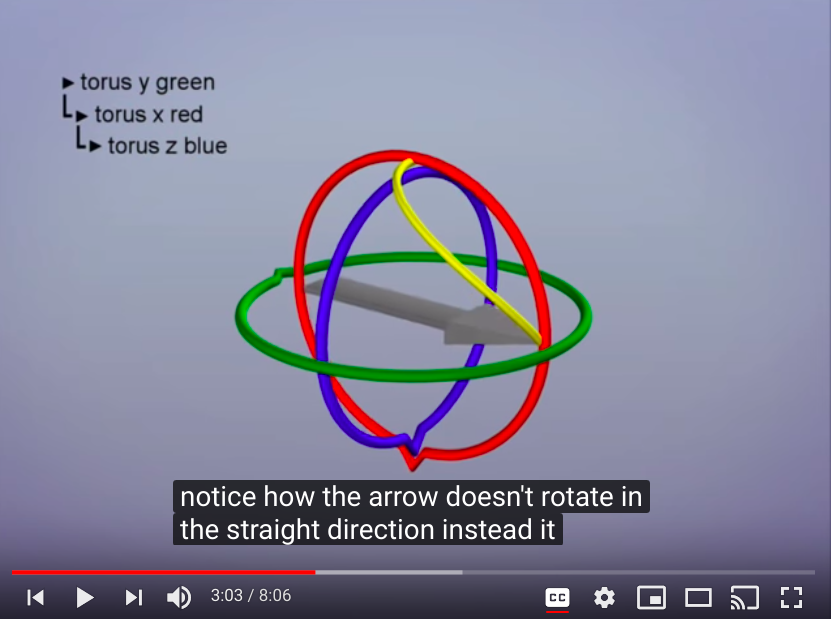It's always possible to rotate our arrow into any new rotation but we have to rotate all three axes to achieve it.

lock之后再做旋转运动的结果就是，end-effector箭头不会按照直线走，而是沿着一个不确定的弧线移动，这样的在两个位置状态之间的移动具有多样性和不确定性，不好控制

• manipulator会失去至少一个自由度，无法实现某些运动
• 加载在某些joint variables（比如角速度，或我理解的比如PWM波驱动舵机）上的改变怎么也不会导致end-effector做出对应的位置和方向改变。要是设计得不好，机器人是不是很容易剧烈运动？即使没有到达奇点位置，运动速度也会突然加快，整体将不稳定
• 无法求逆运算[bib3]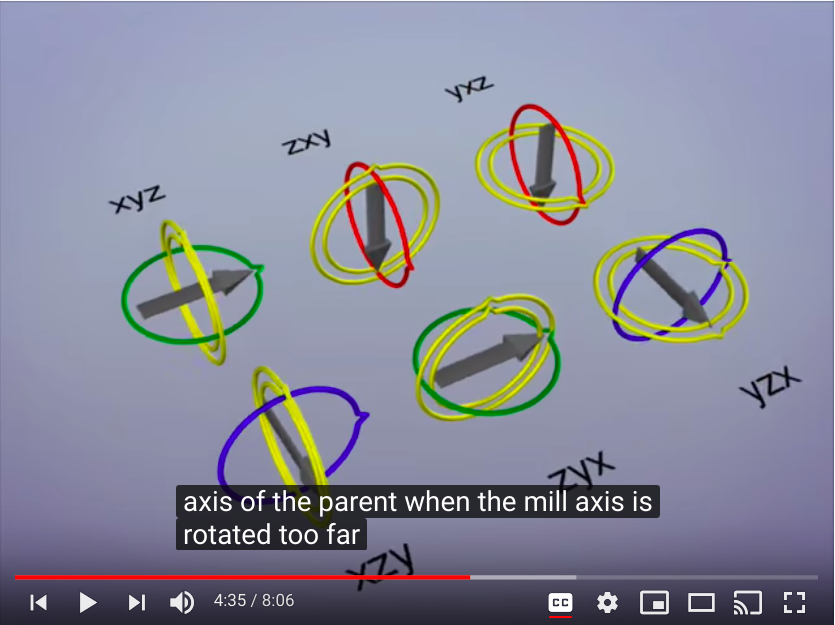With any object, the key if to find a rotation order that has the least chance of hitting gimbal. So the trick here is to find the direction that the object least likely to face.

• 找到最可不常需要面对的位置，将此位置设计为singularity，我们就能避免在其他常规运动时碰到singularity啦！
• 如果确实没办法或者暂时没办法解决，那么在奇点处设计奇点触发保护也是很好的。[bib3]

https://en.wikipedia.org/wiki/Forward_kinematics#cite_note-1

https://en.wikipedia.org/wiki/Orthogonal_matrix

https://www.zhihu.com/question/21966482

rebuilding site 12 Jul 2020 01:09:19